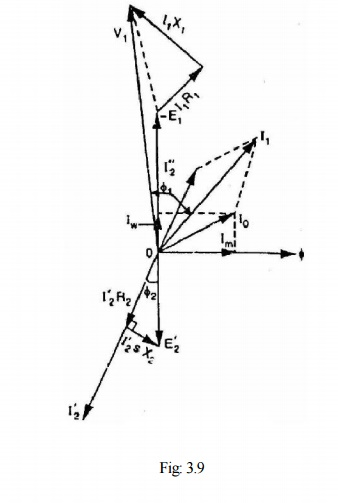Home | | Electrical Machines II | Phasor Diagram of Three Phase Induction Motor

# Phasor Diagram of Three Phase Induction Motor

In a 3-phase induction motor, the stator winding is connected to 3-phase supply and the rotor winding is short-circuited.

Phasor Diagram of Three Phase Induction Motor

In a 3-phase induction motor, the stator winding is connected to 3-phase supply and the rotor winding is short-circuited. The energy is transferred magnetically from the stator winding to the short-circuited, rotor winding. Therefore, an induction motor may be considered to be a transformer with a rotating secondary (short-circuited). The stator winding corresponds to transformer primary and the rotor finding corresponds to transformer secondary. In view of the similarity of the flux and voltage conditions to those in a transformer, one can expect that the equivalent circuit of an induction motor will be similar to that of a transformer. Fig. 3.8 shows the equivalent circuit per phase for an induction motor. Let discuss the stator and rotor circuits separately.Stator circuit. In the stator, the events are very similar to those in the transformer primary. The applied voltage per phase to the stator is V1  and R1and X1  are the stator resistance and leakage reactance per phase respectively. The applied voltage V produces a magnetic flux which links the stator winding (i.e., primary) as well as the rotor winding (i.e., secondary). As a result, self-induced e.m.f. E1  is induced in the stator winding and mutually induced e.m.f.

E'2 (= s E2 = s K E2  where K is transformation ratio) is induced in the rotor winding. The flow of stator current I1 causes voltage drops in R1 and X1.

V1 = - E1 + I1(R1+jX1)  …… phasor sum

When the motor is at no-load, the stator winding draws a current I0. It has two components viz.,

(i) which supplies the no-load motor losses and (ii) magnetizing component Im which sets up magnetic flux in the core and the air gap. The parallel combination of Rc and Xm, therefore, represents the no-load motor losses and the production of magnetic flux respectively.

I0 = Iw + Im

Rotor circuit. Here R and X represent the rotor resistance and standstill rotor reactance per phase respectively. At any slip s, the rotor reactance will be X2 .The induced voltage/phase in the rotor is E'2 = s E = s K E1 . Since the rotor winding is short-circuited, the whole of e.m.f. E'2 is used up in circulating the rotor current I'2.

E’2 = I’2 (R2 + jsX2)

The rotor current I'2 is reflected as I"2 (= K I'2) in the stator. The phasor sum of I"2 and I0 gives the stator current I1.

It is important to note that input to the primary and output from the secondary of a transformer are electrical. However, in an induction motor, the inputs to the stator and rotor are electrical but the output from the rotor is mechanical. To facilitate calculations, it is desirable and necessary to replace the mechanical load by an equivalent electrical load. We then have the transformer equivalent circuit of the induction motor.It may be noted that even though the frequencies of stator and rotor currents are different, yet the magnetic fields due to them rotate at synchronous speed Ns. The stator currents produce a magnetic flux which rotates at a speed Ns. At slip s, the speed of rotation of the rotor field relative to the rotor surface in the direction of rotation of the rotor isBut the rotor is revolving at a speed of N relative to the stator core. Therefore, the speed of rotor field relative to stator coreThus no matter what the value of slip s, the stator and rotor magnetic fields are synchronous with each other when seen by an observer stationed in space. Consequently, the 3-phase induction motor can be regarded as being equivalent to a transformer having an air-gap separating the iron portions of the magnetic circuit carrying the primary and secondary windings. Fig. 3.9 shows the phasordiagram of induction motor.

Study Material, Lecturing Notes, Assignment, Reference, Wiki description explanation, brief detail
Electrical machines : Three Phase Induction Motor : Phasor Diagram of Three Phase Induction Motor |